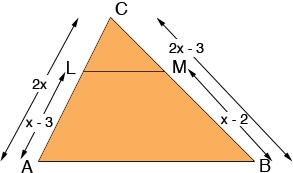SEARCH HOMEMath Central Quandaries & QueriesQuestion from Nisha, a student: In triangle ABC, LM is parallel to AB. If AL=x-3,AC=2x,BM=x-2,BC=2x=3. Find xHi Nisha,

I think you have a diagram that looks likeSince LM is parallel to AB the triangles ABC and LMC are similar. Hence, in particular

|CA|/|BC| = |CL|/|MC|

Thus

2x/(2x - 3) = |CL|/|MC|

Hoe long is CL? How long is MC? substitute these values and solve for x.

PennyMath Central is supported by the University of Regina and The Pacific Institute for the Mathematical Sciences.